Unit Conversions (Part 2):

by on July 18th, 2018In Part 1, we dealt with converting between different metric units. Check that out if you haven’t already.

Converting from one metric unit to another is relatively straightforward since it’s all about powers of ten, but dealing with the Imperial system—feet, miles, inches—is a bit more involved. (Side note: the only countries in the world that don’t use the metric system are the USA, Liberia, and Myanmar. Quite the trio.)

So let’s try another one from GMAT Prep. Two minutes on the clock, and go:

If it took Carlos 1/2 hour to cycle from his house to the library yesterday, was the distance that he cycled greater than 6 miles? (Note: 1 mile = 5280 ft)

(1) The average speed at which Carlos cycled from his house to the library yesterday was greater than 16 feet per second.
(2) The average speed at which Carlos cycled from his house to the library yesterday was less than 18 feet per second.

(A) Statement (1) ALONE is sufficient, but statement (2) alone is not sufficient.
(B) Statement (2) ALONE is sufficient, but statement (1) alone is not sufficient.
(C) BOTH statements TOGETHER are sufficient, but NEITHER statement ALONE is sufficient.
(D) EACH statement ALONE is sufficient.
(E) Statements (1) and (2) TOGETHER are NOT sufficient.

They give us the miles to feet conversion, but if you got stuck on the math, here’s another hint that might help get you unstuck: 5280 = 88 * 60. Take some more time if you need it.

Okay, all done? Let’s see what’s going on here.

First things first: analyze the question stem. When doing Data Sufficiency questions, lots of students read the question and think, “Question, schmestion! Let’s get to the good stuff!” Big mistake. Always start with the question. The more work you do analyzing the question, the easier the statements will be.

If it took Carlos 1/2 hour to cycle from his house to the library yesterday, was the distance that he cycled greater than 6 miles? (Note: 1 mile = 5280 ft)

Let’s take the given information and plug it into the relationship Rate * time = distance.

They give us Carlos’ time and ask if his distance was more than 6 miles, so we can turn this question into an inequality:

“Is Rate * 1/2 hr > 6 miles?”

Keep in mind that it’s still a question, so we still need to put a question mark at the end of it. But we can manipulate and rephrase it just like we would any other inequality—let’s isolate Rate:

“Is Rate > 6 miles / (1/2 hr)?”

And now simplify the right-hand side:

“Is Rate > 12 miles per hour?”

So this question is really asking, “Was Carlos going faster than an average speed of 12 miles per hour?” Now we can start dealing with the statements. Both statements give Carlos’ speed in feet per second. It might be tempting to think, “They ask us for his speed, and then each statement tells us about his speed, so each statement is sufficient.”

But that’s not always going to be the case. For instance, if they told us that Carlos was going faster than 14 miles per hour on average, then yes, we would know that he must have been going faster than 12 miles per hour on average, since 14 mph is faster than 12 mph. But what if they told us that Carlos was going faster than 10 miles per hour on average—then we wouldn’t know whether he was also going faster than 12 mph. So we have to actually do the unit conversions to see what’s going on.

The next step is to determine which way we want to do the conversion. We have two options:

-Convert the question stem from miles per hour into feet per second to match the statements
-Convert the statements from feet per second into miles per hour to match the question stem

Either way will work, but the first is going to be less work since we’ll only have to do the conversion once. If we do it the other way around, we’ll have to convert both statements into miles per hour, so that would be twice as much work.

So let’s go back to the question again and convert 12 miles per hour into feet per second.

Set up our given ratio (12 miles per 1 hour) on the left and our goal ratio (x feet per 1 second) on the right: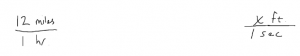Now we need to convert hours to seconds, and convert miles to feet.

There are 60 minutes in 1 hour and 60 seconds in one minute. Since hours is currently in the denominator, I need hours in the numerator of another fraction so they’ll cancel out. Repeat the same process for minutes to seconds.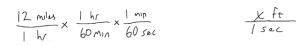To convert miles to feet, use the given information that 1 mile = 5280 feet. And since miles are in the numerator of our given ratio, we need to cancel that out by putting miles in the denominator: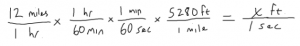Now cancel out hours with hours, minutes with minutes, and miles with miles: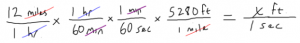Now we’re left with feet over seconds, which is what we want. All that’s left is to do the arithmetic, which looks tricky, but here’s where that 5280 = 88 * 60 comes in handy.(I recommend putting 5280 = 88 * 60 on a flashcard. Or if you’re a fan of 80s movies, I just remember that the Delorean from Back to the Future had to go 88 miles per hour to travel through time.)

Now we can cancel out a pair of 60s in the top and in the bottom, and 12/60 reduces to 1/5: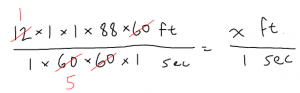We’re left with 88 feet per 5 seconds: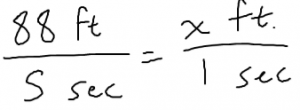88/5 = 17.6, so x = 17.6 feet per second.

So, the original question has now been further rephrased into:

“Was Carlos going faster than an average speed of 17.6 feet per second?”

Now we can compare the (thoroughly rephrased) question with the statements. Statement 1:

(1) The average speed at which Carlos cycled from his house to the library yesterday was greater than 16 feet per second.

Okay, he was going faster than 16 f/s, but that doesn’t tell us whether he was going faster than 17.6 f/s. Maybe he was going 16.1 f/s, or maybe he was going 20 f/s—either is possible, and one is slower than 17.6 while the other is faster. Insufficient—that eliminates answers A and D.

Moving on to Statement 2 (and pretending that we don’t know the information from Statement 1):

(2) The average speed at which Carlos cycled from his house to the library yesterday was less than 18 feet per second.

So we know he was going slower than 18 f/s, but that still doesn’t tell us whether he was going faster than 17.6 f/s. Insufficient—that eliminates answer B.

If we put both statements together, we know that he was going faster than 16 f/s and slower than 18 f/s, but 17.6 is in between the two so we still don’t know if he was going faster or slower than 17.6. The answer is E—both statements together are not sufficient to answering the question.